# How to Solve One-Step Equations with Fractions

Instructor: Laura Pennington

Laura received her Master's degree in Pure Mathematics from Michigan State University. She has 15 years of experience teaching collegiate mathematics at various institutions.

This lesson will explain how to solve one step equations with fractions. We will define one step equations, then work with fractions to solve these types of equations, and finally look at a couple of applications involving these equations.

## Steps to Solve

When it comes to solving one step equations, the number of steps we take is exactly the number that the name implies - one! One step equations are equations that involve adding, subtracting, multiplying, or dividing both sides of the equation by the same number, variable, or term in order to isolate and solve for the variable.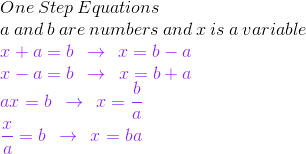We see that to solve one step equations, we simply add, subtract, multiply, or divide the same number from both sides to solve for the unknown variable. Therefore, in order to solve one step equations with fractions, we simply need to know how to add, subtract, multiply, and divide fractions, so let's have a quick refresher on the rules for these operations on fractions.

To add or subtract fractions, we find a common denominator, add or subtract numerators, and then simplify the result. We can use the following rule to add or subtract fractions.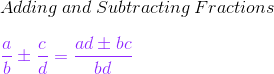Surprisingly, multiplying and dividing fractions is easier than adding and subtracting fractions, especially multiplying fractions. To multiply fractions, we simply multiply the numerators, multiply the denominators, and then simplify the result.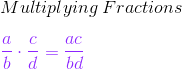Pretty straightforward, wouldn't you say? Lastly, to divide fractions, we simply turn it into a multiplication problem by multiplying the numerator by the reciprocal of the denominator, where the reciprocal of a fraction is obtained by interchanging the numerator and denominator.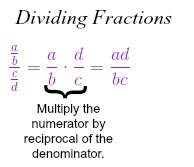Okay, now that we've had a refresher on performing arithmetic operations on fractions, we can solve one step equations involving fractions using one step. That is, isolate the variable on one side of the equation by adding, subtracting, multiplying, or dividing a fraction from both sides of the equation. To determine which operation to use, we just use the opposite operation of the operation that is being used in the original equation. The following table illustrates this concept.

Original Equation Isolated Variable Operation Used
x + a = b x = b - a Subtract a from both sides.
x - a = b x = b + a Add a to both sides.
ax = b x = b / a Divide both sides by a.
x / a = b x = ba Multiply both sides by a.

## Solution

To solve one step equations involving fractions, we add, subtract, multiply, or divide a fraction from both sides of the equation to isolate the variable. That is, if a and b are fractions, and x is a variable, then we use the rules in the following table to solve for x in each of the one step equations.

Original Equation Isolated Variable Operation Used
x + a = b x = b - a Subtract a from both sides.
x - a = b x = b + a Add a to both sides.
ax = b x = b / a Divide both sides by a.
x / a = b x = ba Multiply both sides by a.

## Applications

We don't know about you, but when it comes to learning mathematical processes, but many people can read steps, facts, and rules all day, but until they put them into practice, it's difficult to retain that information. Therefore, let's look at a couple of examples of applying this solving process!

First of all, suppose you and a friend are going for a bike ride. The two of you are planning on riding for 8 2/3 miles (or 26/3 miles). We've already gone 4 5/6 miles (or 29/6 miles), and we want to figure out how much further we have to go. If we call the distance we have left to go x, then it must be the case that x + 29/6 = 26/3.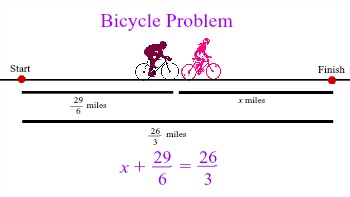To unlock this lesson you must be a Study.com Member.

### Register to view this lesson

Are you a student or a teacher?

#### See for yourself why 30 million people use Study.com

##### Become a Study.com member and start learning now.
Back
What teachers are saying about Study.com

### Earning College Credit

Did you know… We have over 200 college courses that prepare you to earn credit by exam that is accepted by over 1,500 colleges and universities. You can test out of the first two years of college and save thousands off your degree. Anyone can earn credit-by-exam regardless of age or education level.